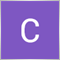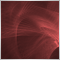# How to convert Negative to positive vales (absolute values) in Mql519

Hi guys.
Im kinda new to coding EAs in Mql5.
But i am trying to get the absolute values of variables, mainly my 'Loss'. I know that the MathAbs() function can solve the problem but i don't know how to execute it.
Here is where i am at:
double Loss = (1 + total pips for loss)
double MathAbs (
double Loss
) ;

I know that me putting the loss there is wrong, but i really don't know how to go about getting the numeric value for this.5043

Clinton Okechwuku:
Hi guys.
Im kinda new to coding EAs in Mql5.
But i am trying to get the absolute values of variables, mainly my 'Loss'. I know that the MathAbs() function can solve the problem but i don't know how to execute it.
Here is where i am at:
double Loss = (1 + total pips for loss)
double MathAbs (
double Loss
) ;

I know that me putting the loss there is wrong, but i really don't know how to go about getting the numeric value for this.

```double yourValue  = -500.24;23417

Clinton Okechwuku: I know that the MathAbs() function can solve the problem b

Perhaps you should read the manual.
How To Ask Questions The Smart Way. 2004